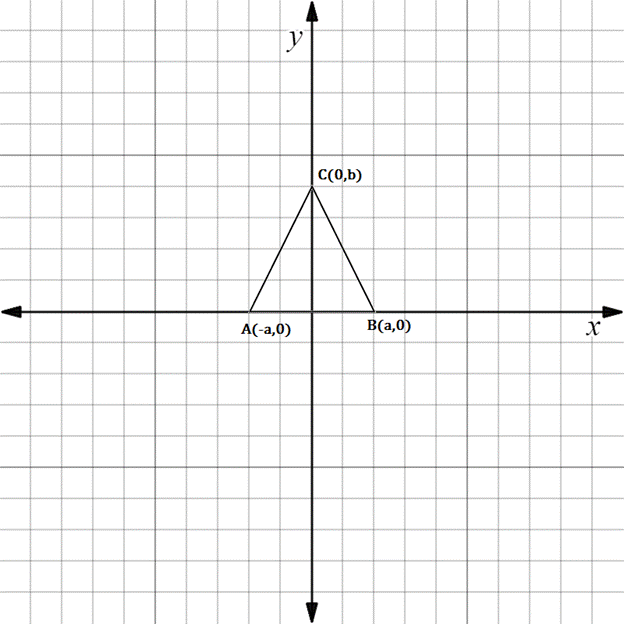Chapter 10.3, Problem 29E### Elementary Geometry for College St...

6th Edition
Daniel C. Alexander + 1 other
ISBN: 9781285195698

#### Solutions

Chapter
Section### Elementary Geometry for College St...

6th Edition
Daniel C. Alexander + 1 other
ISBN: 9781285195698
Textbook Problem
14 views

# The drawing shows isosceles △ A B C with A C - ≅ B C - .a) What type of number is a ?b) What type of number is – a ?c) Find an expression for the length of A B - .To determine

(a)

To find:

The type of number a using the given isosceles triangle.

Explanation

The given figure is shown below.

To determine

(b)

To find:

The type of number -a using the given isosceles triangle.

To determine

(c)

To find:

The expression for the length AB-.

### Still sussing out bartleby?

Check out a sample textbook solution.

See a sample solution

#### The Solution to Your Study Problems

Bartleby provides explanations to thousands of textbook problems written by our experts, many with advanced degrees!

Get Started

#### Simplify the expressions in Exercises 97106. x3/2x5/2

Finite Mathematics and Applied Calculus (MindTap Course List)

#### For

Study Guide for Stewart's Multivariable Calculus, 8th

#### cosh 0 = a) 0 b) 1 c) e22 d) is not defined

Study Guide for Stewart's Single Variable Calculus: Early Transcendentals, 8th